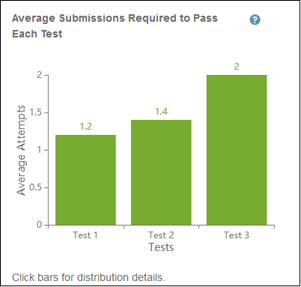## View Progress with Class Overview

Learner Analytics provide a way to assess learner progress using visual graphs. To access Learner Analytics, you must have a paid MATLAB® Grader™ license. See View Options under Get Started at MATLAB Grader for more information on features and license requirements.

To view these graphs, go to any problem and then click Class Overview.

### Status SummaryThis graph shows the percentages of learners who have:

• Solved the problem.

• Not solved the problem but have made at least one submission.​

The percentages represent learners who are in the course.

### Submissions Required to Solve the Problem

This graph shows the number of submissions required to solve the problem.Key points:

• The graphs represent only learners who have solved the problem.

• The percentages represent the number of submissions it took per learner to submit a solution that solves the problem. The first solution that solves the problem is counted. For example, if a learner solves the problem in their third submission, then three submissions for that learner are counted, even if the learner continues to submit additional solutions, correct or not.

• If the assessment method is weighted, the problem is considered solved only when the learner’s score is 100%.

### Average Submissions Required to Pass Each Test

This graph shows the average number of submissions required for learners to pass each test.​For descriptions of each test and distribution details, click on the test bar.

Key points:

• The graph represents only learners who have solved the problem.

• The average for each test reflects when a learner permanently passes a test. For example, the learner passes a test for the first time on the second submission, fails it on the third and fourth submissions, and then passes it again on the fifth submission. If the learner continues to pass the test in subsequent submissions, MATLAB Grader records five as the number of times required to pass that test.

### Submissions Made Attempting to Solve the Problem

This graph shows the number of submissions learners have made attempting to solve the problem, by percentage of learners.Key points:

• The percentages are of learners who have not yet solved the problem but have made at least one submission.​

• If the assessment method is weighted, the problem is considered solved only when the learner’s score is 100%.

### Learners Still Failing Each Test

This graph shows the percentage of learners still failing each assessment test.• ​The graph distinguishes between the following types of errors:

• Assertion–The test condition failed. For example, if a test checks whether variable x equals the reference solution, but it does not, the test fails.

• Runtime–An error condition occurred when executing the code, requiring execution to stop. Possibilities include calling a MATLAB function with incorrect input parameters, negative indexing of a vector variable, or assigning an undefined function or variable. For script-based problems, runtime errors result in all tests failing.

• Syntax–The code contains a syntax error. For both script-based and function-based problems, syntax errors result in all tests failing.

Key points:

• The percentages are of learners who have not yet solved the problem but have made at least one submission.​

• The graph reflects each learner’s most recent submission. For example, if a learner passed Test 2 in a previous submission but failed in their most recent submission, the graph counts the learner as failing the test.